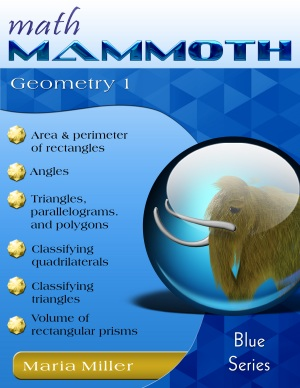You are here: HomeOnline resourcesGeometry → Angles

# Angles: online games, activities, and lessons

This is an annotated and hand-picked list of online games, activities, and lessons for angle-related topics in elementary school.

## Angles

Guess the Random Angle
Estimate and guess the angles shown.
www.crickweb.co.uk/assets/resources/flash.php?&file=angle

Guide the ladybug by giving her commands to turn 90° or 45°, right or left, or to move forward/backward.
nlvm.usu.edu/en/nav/frames_asid_287_g_2_t_3.html

Similar to the Ladybug Leaf, but this time you guide the ladybug through the maze.
nlvm.usu.edu/en/nav/frames_asid_141_g_2_t_3.html

Angles
Practice your knowledge of acute, obtuse, and alternate angles, plus relationships between angles such as vertical, adjacent, alternate, same-side, and corresponding.
www.shodor.org/interactivate/activities/Angles

Angle Find!
Click on the angle with the given angle measure within a geometric figure. Three different modes: Easy Cornering, Parallel Play, and Tangled Angles. Practices your ability to estimate angles and your knowledge of vertical angles, corresponding angles, and angles in a triangle.
puzzlezapper.com/aom/mathed/anglefind/anglefind.html

#### Key to Geometry Workbooks

Here is a non-intimidating way to prepare students for formal geometry. Key to Geometry workbooks introduce students to a wide range of geometric discoveries as they do step-by-step constructions. Using only a pencil, compass, and straightedge, students begin by drawing lines, bisecting angles, and reproducing segments. Later they do sophisticated constructions involving over a dozen steps-and are prompted to form their own generalizations. When they finish, students will have been introduced to 134 geometric terms and will be ready to tackle formal proofs.

Geometry Bridge
An interactive review lesson on types of angles, types of triangles, angle sum of a triangle, and the Pythagorean Theorem, for 5th-7th grade students. You get to build a bridge!
www.sharemylesson.com/teaching-resource/Geometry-Bridge-50018309/

Turtle Pond
Estimate length and angle measure while guiding a turtle to a pond using computer commands.
illuminations.nctm.org/Activity.aspx?id=3534

Banana Hunt at Primary Games
Help the monkey to find bananas and learn to estimate angles.
www.primarygames.co.uk/pg2/bhunt/bhunt.html

Angles
Practice obtuse/right/acute angles, and vertical/corresponding/alternate interior or exterior angle concepts
www.shodor.org/interactivate/activities/AnglesMath Mammoth Geometry 1
A self-teaching worktext for 4th-5th grades that covers angles, triangles, quadrilaterals, circle, symmetry, perimeter, area, and volume. The book contains lots of hand-son drawing exercises. Available as a download version (\$6.90) and a printed copy.
www.mathmammoth.com/geometry_1.php

Working with Angles
An interactive lesson with explanations and quiz from Absorb Mathematics course written by Kadie Armstrong, a mathematician.
www.absorblearning.com/mathematics/demo/units/KCA003.html

Angles in Polygons
An interactive lesson with explanations and quiz from Absorb Mathematics course written by Kadie Armstrong, a mathematician.
www.absorblearning.com/mathematics/demo/units/KCA004.html

Properties of Parallelograms
Investigate the sides and interior angles of parallelograms using these two interactive tools.
math.kendallhunt.com/x19430.html

Interior Angles
A nice lesson and explanation about interior angles of polygons.
www.coolmath4kids.com/interior.htmlDr. Math Introduces Geometry: Learning Geometry is Easy! Just ask Dr. Math!
A supplement to middle school geometry, Dr. Math Introduces Geometry has a light tone, clear layout, and humorous cartoons by Jessica Wolk-Stanley. The best part is that the explanations to math questions are accurate and clear. You'll find answers to dozens of real questions from students who needed help understanding the basic concepts of geometry. This book covers angles, triangles, quadrilaterals, area, perimeter, Pi, circle parts, polyhedra, surface area, volume, nets, congruent transformations, and symmetry. Price: about \$12 new, \$1-\$6 used.

Geometry worksheets & quizzes
Worksheets about complementary and supplementary angles, parallel, perpendicular, and intersecting lines, types of angles, basic shapes, area & perimeter of rectangles, and parts of a circle.

MB-Ruler - a triangular ruler for the computer screen
MB-Ruler is a software that helps you to measure distances and angles on the computer screen, such as distances on a map or angles in any image. It has a ruler and a protractor, which are almost transparent over the image you are working with.